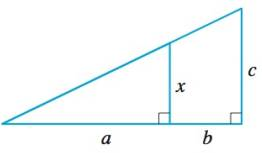Chapter A, Problem 13E### Algebra and Trigonometry (MindTap ...

4th Edition
James Stewart + 2 others
ISBN: 9781305071742

#### Solutions

Chapter
Section### Algebra and Trigonometry (MindTap ...

4th Edition
James Stewart + 2 others
ISBN: 9781305071742
Textbook Problem

# 13-14 Using Similarity Express x in terms of a, b, and c.To determine

To express:

The x in terms of a,bandc.

Explanation

Given:

The given pair of triangles is similar.

The given pair of triangle is,

Figure (1)

Approach:

Two triangles are similar if their vertices can be matched up so that corresponding angles are congruent. In this case corresponding sides are proportional.

Calculation:

By similarity,

The lengths of the corresponding sides in the triangles are proportional.

### Still sussing out bartleby?

Check out a sample textbook solution.

See a sample solution

#### The Solution to Your Study Problems

Bartleby provides explanations to thousands of textbook problems written by our experts, many with advanced degrees!

Get Started